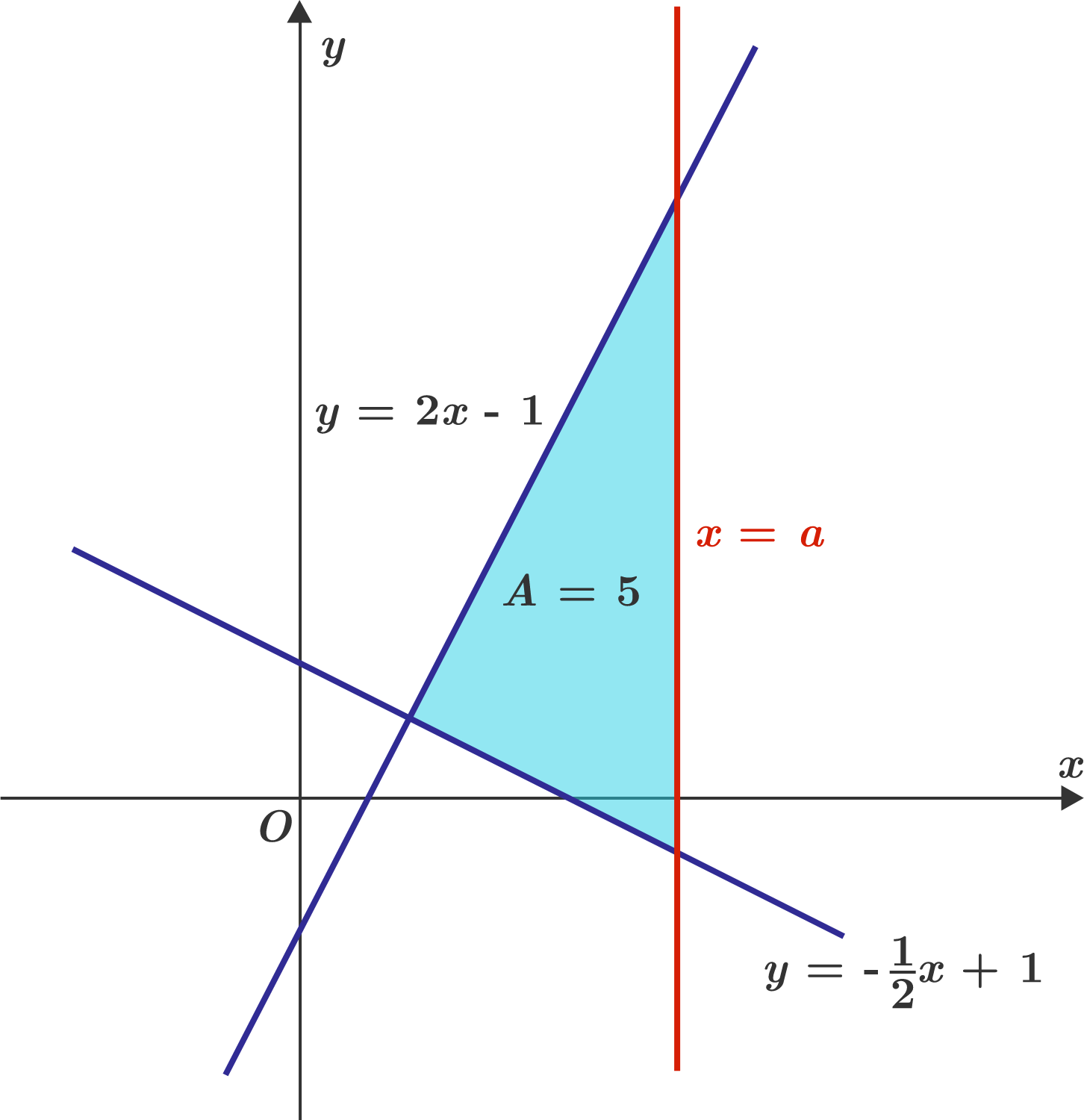# Bounding A Triangle

Geometry Level 4For $a> 0$, the above diagram shows the vertical line $x=a$ bounds a triangle with the lines $y=2x-1$ and $y = -\frac x2 + 1$.

If the constraint $a>0$ is relaxed, then there is another possible value of $a$ such that the enclosed area of the new triangle is also 5.

If the sum of these two values of $a$ can be expressed as $\dfrac BC$, where $B$ and $C$ are coprime positive integers, find $B+C$.

×